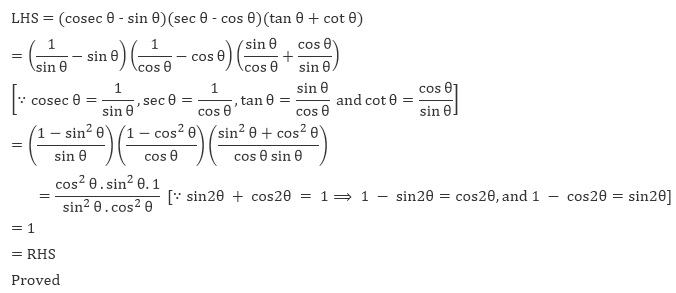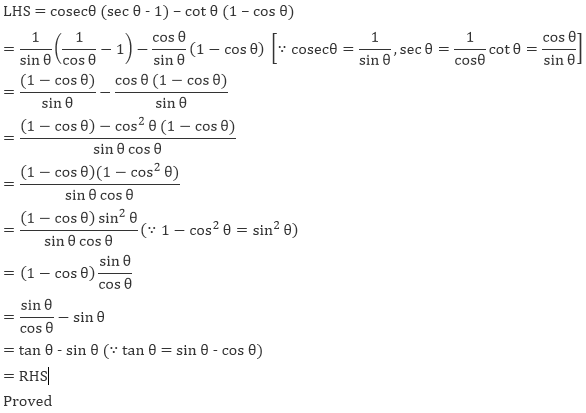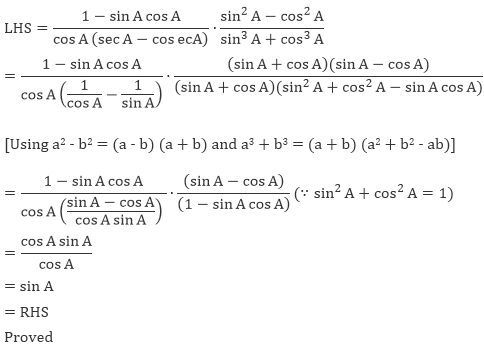#### Thank you for registering.

One of our academic counsellors will contact you within 1 working day.

Click to Chat

1800-5470-145

+91 7353221155

CART 0

• 0
MY CART (5)

Use Coupon: CART20 and get 20% off on all online Study Material

ITEM
DETAILS
MRP
DISCOUNT
FINAL PRICE
Total Price: Rs.

There are no items in this cart.
Continue Shopping

# Chapter 5: Trigonometric Functions – Exercise 5.1

## Trigonometric Functions – Exercise 5.1 – Q.1

LHS = sec4 θ - sec2 θ

= sec2 θ(sec2 θ -1)

= (1 + tan2 θ) tan2 θ     [∵ sec2 θ = 1 + tan2 θ]

= tan2 θ + tan4 θ

= tan4 θ + tan2 θ

= RHS

LHS = RHS

Proved

## Trigonometric Functions – Exercise 5.1 – Q.2

LHS = sin6 θ + cos6 θ

= (sin2 θ)3 + (cos2 θ)3

= (sin2 θ + cos2 θ)[(sin2 θ)2 - sin2 θ cos2 θ + (cos2 θ)2]               (∵ a3 + b3 = (a + b)(a2 - ab + b2))

= (sin2 θ)2 + (cos θ)2 + 2 sin2 θ cos2 θ - 2sin2 θ cos2 θ - sin2 θ = sin2 θ cos2 θ)

[adding and subtracting 2 sin2 θ cos2 θ and using identity sin2 θ + cos2 θ = 1]

= (sin2 θ + cos2 θ)2 - 3 sin2 θ cos2 θ

= (sin2 θ + cos2 θ)2 - 3 sin2 θ cos2 θ

= 12 - 3 sin2 θ cos2 θ (∵ sin2 θ + cos2θ = 1)

= 1 - 3 sin2 θ cos2 θ

= RHS

∴ LHS = RHS

Proved

## Trigonometric Functions – Exercise 5.1 – Q.3## Trigonometric Functions – Exercise 5.1 – Q.4## Trigonometric Functions – Exercise 5.1 – Q.5## Trigonometric Functions – Exercise 5.1 – Q.6## Trigonometric Functions – Exercise 5.1 – Q.7## Trigonometric Functions – Exercise 5.1 – Q.8

LHS = (sec A sec B + tan A tan B)2 - (sec A tan B + tan A sec B)2

= (sec A sec B)2 + (tan A tan B)2 +2sec A sec B tan A tan B - ((sec A tan B)2 + (tan A tan B)2 + 2sec A tan B tan A sec B) [using (a + b)2 = a2 + b2 + 2ab]

= sec2 A sec2 B + tan2 A tan2 B + 2sec A sec B tan A tan B - sec2 A tan2 B - tan2 A sec2 B -  2sec A sec B tan A tan B [using (ab)2 = a2b2]

= sec2 A sec2 B - sec2 A tan2 B + tan2 A tan2 B - tan2 A sec2 B

= sec2 A(sec2 B - tan2 B)+ tan2 A(tan2 B - sec2 B)

= sec2 A 1 - tan2 A1 [∵ sec2 θ = 1 + tan2 θ ⟹ sec2 θ - tan2 θ = 1]

= 1 + tan2 A - tan2 A

= 1

= RHS

Proved

## Trigonometric Functions – Exercise 5.1 – Q.9## Trigonometric Functions – Exercise 5.1 – Q.10